Wednesday, July 25, 2012 ...//Euler characteristic

Topology is an important branch of mathematics that studies all the "qualitative" or "discrete" properties of continuous objects such as manifolds, i.e. all the properties that aren't changed by any continuous transformations except for the singular (infinitely extreme) ones.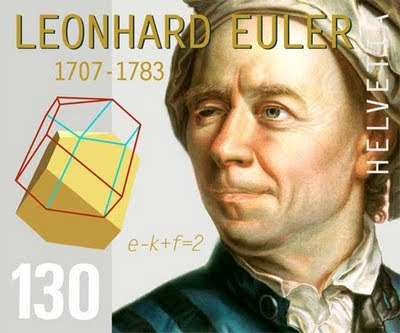In this sense, topology is a vital arbiter in the "discrete vs continuous" wars. The very existence of topology as a discipline shows that "discrete properties" always exist even if you only work with continuous objects. On the other hand, topology always assumes that these features are "derived" – they're some of the properties of objects and these objects are deeper and that may have many other, continuous properties, too. The topological, discrete properties of these objects are just projections or caricatures of the "whole truth".

The sphere – the surface of a ball – can't be continuously deformed to a torus – the idealized two-dimensional inner tube inside a tire. The torus has a hole in the middle. So they're topologically distinct two-dimensional manifolds. We may prove that they're topologically different if we find a "topological invariant" – a number or a more complicated quantity that doesn't change by any continuous deformations – that has different values for both manifolds. Of course, the "number of holes" (known as genus) is a way to distinguish a sphere from a torus.

If a topological invariant coincides for two manifolds, they may still be topologically different because there may exist other kinds of topological invariants that differ. (And if we're unlucky, their difference may even boil down to invariants that are unknown right now; in that case, it may be hard to distinguish them.) So "the number of holes" is just one possible invariant. But we will talk about "the number of holes" in this article and generalize it to a more natural quantity, the Euler characteristic (or "Euler number" if you're among topologists or topologians – let's hope that they differ less than physicists differ from physicians: otherwise "Euler number" is being used for other things which still differ from the Euler constant and many other things named after this emperor of mathematicians).

I instinctively use the term "Euler character" but I have carefully corrected the term to "Euler characteristic" throughout this text, a term which is probably more accurate. ;-)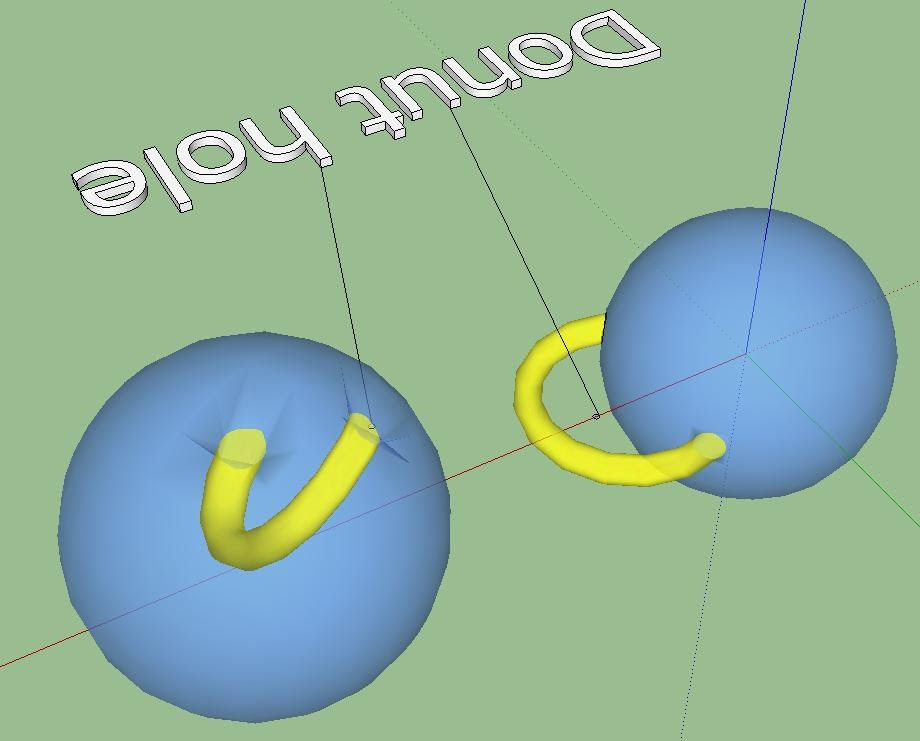A torus is topologically equivalent to a (blue) sphere with a (yellow) handle. Just cut two small disks into the sphere and connect them with a tube. The resulting surface is topologically equivalent (diffeomorphic) to a torus, as you may see by gradually deflating the (blue) balloon. Of course, you may also create more complicated shapes by adding $$h$$ handles instead of one handle. Just to be sure, only the surfaces are counted, not the "bulk". The surface with $$h$$ handles is known as the genus $$h$$ Riemann surface.A genus-2 Riemann surface

Instead of the number of holes $$h$$, we will describe the same information in terms of the Euler characteristic $$\chi$$ – the Greek letter is pronounced by English speakers as "chi" i.e. "kchaj" in the Czech transliteration even though it should be pronounced as the Czechs do, namely as "khee" i.e. "chí":$\chi=2-2h.$ If you determine $$h$$, you know $$\chi$$, and vice versa. You might think that $$\chi$$ is more artificial but we will see many formulae for $$\chi$$ that show that it is actually more natural a label distinguishing manifolds than $$h$$ itself. The first such formula is one for polyhedra:$\chi = V - E + F.$ We just sum (or subtract) the number of vertices $$V$$, the number of edges $$E$$, and the number of faces $$F$$ with alternating signs. If we had a manifold whose dimension would exceed two (recall that we're talking about the surface of the polyhedron only), the formula would continue to hold:$\chi = \sum_{n=0}^d (-1)^n E_n$ where $$E_n$$ generalizes the number of $$n$$-dimensional "edges" which may be vertices, edges, faces, hyperfaces, or user-friendly interfaces as the dimensions increase from zero. ;-)

I forgot to tell you that the formula $$\chi=2-2h$$ is equal to $$2$$ for a sphere, $$h=0$$. So if our new vertices-edges-faces formula is any good, you should get $$2$$ for any polyhedron that has no hole, whether it is regular or not. In particular, Platonic polyhedra have no holes so they should give you $$\chi=2$$. Let's check it.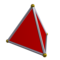Tetrahedron: $$\chi = 4 - 6 + 4 = 2$$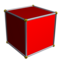Hexahedron (cube): $$\chi = 8 -12 + 6 = 2$$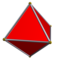Octahedron: $$\chi = 6 -12 + 8 = 2$$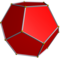Dodecahedron: $$\chi = 20 - 30 + 12 = 2$$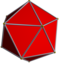Icosahedron: $$\chi = 12 - 30 + 20 = 2$$

If the names of the objects sound Greek to you, then it is correct because the names come from Greek. More importantly, the result is equal to two in all cases.

If you've never noticed that, pairs of the Platonic polyhedra are related: the dual partner has the same number of edges but the numbers of vertices and faces are interchanged. The icosahedron is dual to the dodecahedron; the cube is dual to the octahedron; the tetrahedron is dual to itself. The duality goes beyond this numerology: you may obtain the dual polyhedron by replacing the faces of the original one with vertices and connecting them. The new edges will then just intersect the old ones so their numbers will match.

Off-topic but somewhat cool. Pilsner Catholic bishop Mr František Radkovský (*1939) recorded this song (the narrator is himself) in order to collect some money for the bells at the Pilsner Tower, the St Bartholomew Cathedral. Mr Radkovský is a graduate of my Alma Mater, the Faculty of Mathematics and Physics of the Charles University in Prague, specialization mathematical statistics.

It is not a coincidence that the alternating sum yielded $$2$$ in all cases. You may rather easily prove that the alternating sum is invariant under all combinatorial changes of the polyhedron that emulate the continuous deformations. For example, $$\chi$$ doesn't change if you add a new vertex inside a face and connect it with all the $$k$$ vertices of the original face, a polygon or $$k$$-gon, by $$k$$ new edges. By this procedure, you increase the number of faces by $$k-1$$, from $$1$$ to $$k$$, the number of vertices by one, so the positive terms increase by $$k$$, but you also added $$k$$ edges which are subtracted so the alternating sum is unchanged. In the same way, you may divide an edge, or you may do these procedures backwards. It just works.

You are also invited to check that $$\chi=0$$ for a cubist, Picasso version of a torus.Yup, because of the white background of these pictures, the mobile template prettifies this article a bit.

If we divide the faces to the smaller ones as indicated on the picture (and we should because the alternating formula shouldn't include any faces with holes: the separation of the surface should be fine enough to obey this condition), we find out that this "cuboid torus" has $$32$$ faces (8 upper, 8 lower, 4 inside, 12 outside), $$32$$ vertices (an upper grid and a lower grid with $$4\times 4$$ vertices), and $$64$$ edges (96 edges from 8 little cubes per 12 edges, but 32 have been double-counted on the 8 interfaces of the little cubes per 4). In total,$\chi = 32+32-64 = 0.$ That agrees with $$\chi=2-2h$$ for $$h=1$$ hole. If you think about it, it must always agree, for arbitrary polyhedra of arbitrary topology, because we have proved that the alternating sum formula is topologically invariant and correctly decreases by two if we add a handle.

A sphere with $$h$$ handles is the most general closed (boundary-less) orientable manifold. But you can also have manifolds with boundaries and unorientable manifolds. Much like the genus $$h$$ surface could have been obtained by adding handles to the sphere, the most general Riemann surface is obtained by adding $$b$$ circular boundaries to the sphere (with handles etc.) and $$c$$ circular crosscaps.

A circular boundary is simply created by cutting a disk: the newborn boundary is circular. A crosscap is the same thing except that we identify the opposite points of the newly created circular boundary. This is indicated by an "X" (therefore "cross" in the name) in the circle (the two lines in the "X" indicate two such identifications of points but we must identify them in all directions). And the impact of such an identification is that the resulting manifold remains closed (boundary-less) because the boundaries were turned into teleportation gates into a new portion of the manifold; however, as you can check by sending a letter to the gate, the orientation is flipped so whenever $$c$$ is positive, the resulting manifold is unorientable.

The disk itself may be thought of as a sphere with one hole/boundary (cut the Northern Hemisphere from the surface of the globe, a disk-shaped hole, and another disk, the Southern Hemisphere, will remain there), i.e. $(h,b,c) = (0,1,0)$ and its Euler characteristic must be $$1$$ because two disks may be interpreted as hemispheres and connected into a $$\chi=2$$ sphere via their shared $$\chi=0$$ circular equator. Relatively to the sphere, the Euler characteristic decreased by one so the formula must be$\chi = 2 - 2h -b \dots$ if $$c=0$$. Similarly, the manifold with $(h,b,c) = (0,0,1)$ is the so-called projective sphere, $$S^2/\ZZ_2$$, which may be obtained by identifying the opposite points on a two-sphere. Imagine that the antipodeans are actually identical to you and your kin. This identification changes the maps of countries to their "mirrors" (the longitude is just shifted by 180 degrees, a rotation, but the latitude is turned upside-down which is the source of the mirroring) so the resulting quotient of the sphere is unorientable. Again, the manifold looks like one-half of a sphere, up to possible subtleties at an equator which has $$\chi=0$$ anyway, so the full formula for the Euler characteristic has to be$\chi = 2 - 2h - b - c.$ From the Euler characteristic of the sphere, $$\chi=2$$, we subtract twice the number of handles, once the number of boundaries, once the number of crosscaps, and that's it. (The fact that the coefficient in front of $$h$$ is twice as large as the coefficient in front of $$b$$ is related to the fact that the closed string coupling constant in string theory is the square of the open string coupling constant.) Let me mention some simple and well-known surfaces. (All these surfaces appear as "Feynman diagrams" i.e. histories of merging and splitting strings in string theory. Closed strings are always there; if they're unorientable, we must also sum over unorientable surfaces. If we also include open strings, we must add open surfaces.)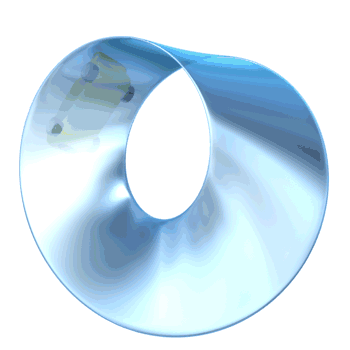Examples of unorientable surfaces

The kids' most favorite unorientable surface is the Möbius strip. You take a strip and glue it to itself after you rotate a short interval at the end by 180 degrees. If a letter "p" swims around such a (transparent) Möbius strip, it becomes a "q", its mirror image. The Möbius strip has a single circular boundary because the potential two original boundaries are twisted into one another. So it must be that $$b=1$$. However, it is unoriented so $$c$$ is positive, too. Well, we have $$c=1$$. There are no handles. We have $\chi = 2 - 1 - 1 = 0.$ Much like the torus, the Möbius strip has a vanishing Euler characteristic. Surfaces with a vanishing Euler characteristic may be visualized as pieces of a flat space with simple identifications. For a torus, it boils down to torus' being a rectangle with the identification of opposite edges. A cylinder is a torus divided by a $$\ZZ_2$$ which acts as a simple left-right reflection with respect to the vertical axis. On the torus, this action has two lines of fixed points which become the cylinder's boundaries. A Klein bottle is another $$\ZZ_2$$ "orbifold" of the torus in which the $$\ZZ_2$$ transformation combines the same left-right reflection we had for the cylinder and a translation by one-half of the torus periodicity in the vertical direction so that there are no fixed points.

As the previous sentence leaked, there exists a simple boundary-less, closed unorientable surface i.e. closed cousin of the Möbius strip called the Klein bottle with $$(h,b,c)=(0,0,2)$$. Melt a bottle of Pilsner Urquell so that it may be easily deformed. Cut a small disk at the bottom. Now drag the neck at the top, bend it down, and attach it to the hole at the bottom. (You must send the neck along a small detour in a fourth dimension of space to avoid a collision i.e. to deal with the tiny technical problem that glass [the travelling neck] can't penetrate another piece of glass [the body of the bottle].)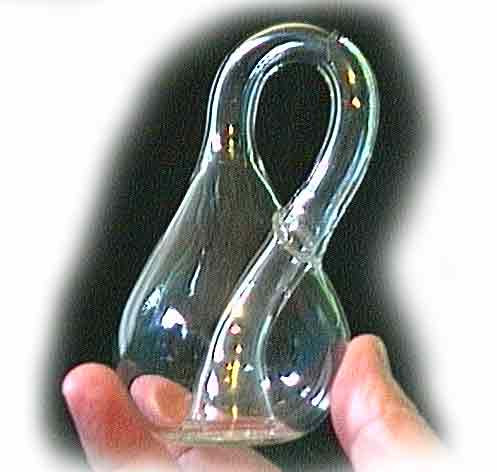Click the picture to learn how to buy this eine kleine Kleinsche Flasche for $35. In the middle of the product, they probably cheated and made the glass self-intersect so you should get a$35 refund.

As emerging (and adult) string theorists know, the Möbius strip with $$(h,b,c)=(0,1,1)$$ is really the geometric average between the Klein bottle and the cylinder (the latter is just a non-Möbius strip, i.e. just a sphere with two boundaries, $$(h,b,c)=(0,2,0)$$). This fact is behind the fact that $$SO(32)$$ type I string theory is free of anomalies.

Aside from the sphere, the torus, the disk, the cylinder, the projective sphere, the Klein bottle, and the Möbius strip, all the other Riemann surfaces are just "some other more complicated manifolds" with various values of $$(h,b,c)$$.

Euler characteristic as an integral of Euler density

The Euler characteritic may also be calculated as an integral of the Euler density which is a function of curvature.$E_{2n} = \frac{1}{2^n} R_{i_1 j_1 k_1 l_1} \dots R_{i_n j_n k_n l_n} \epsilon^{i_1 j_1 \dots i_n j_n} \epsilon^{k_1 l_1 \dots k_n l_n}.$ I suspect some powers of $$1/\pi$$ should be added as well but roughly speaking,$\chi = \int \dd^n x\sqrt{\abs{g}} \, E_{2n}.$ Note that the formula for the Euler density contains $$2n$$ indices in the epsilon symbols; $$n$$ is the dimension of the manifold. These $$2n$$ indices are contracted against those in the Riemann tensor which has $$4$$ indices per piece, and thus we have to include $$n/2$$ copies of the Riemann tensor. The formula only works in the even dimensions. I will later explain what's simple or boring about the Euler characteristic in odd dimensions.

The equivalence of the Euler-density-based formula with the vertices-edges-faces formula may be established by noticing that the Euler-density formula is topologically invariant, too. That's because it's locally a "total derivative". And then one may verify that they produce the same thing if we add handles etc. Well, there are probably better ways to derive the equivalence of the formulae.

For $$n=4$$, the Euler density coincides with the Gauss-Bonnet term and – much like other topological invariants – it doesn't affect the equations of motion (because the equations of motion are derived from infinitesimal variations of the fields but the topological invariant terms in the action don't vary in such a situation). In higher dimensions, however, the same quadratic formula in the curvature tensor is no longer topologically invariant and matters.

An even greater simplification occurs for $$n=2$$ dimensions. We have said that the Riemann surfaces are specified by $$(h,b,c)$$. For boundaries, we would have to add an integral of an extrinsic curvature-based term over the boundary to get the right topologically invariant Euler characteristic (otherwise the integral is not even topologically invariant). In two dimensions, all the components of the Riemann tensor are proportional to the Ricci scalar so the Euler characteristic of closed surfaces is just proportional to the integral of $$R$$ over the manifold (with the proper volume measure).

That's great because we see that it vanishes for a torus which may be given a flat metric: a torus is a flat rectangle with the identification of upper-and-lower edges and the left-and-right edges. Also, for a sphere, $$R=2/a^2$$ where $$a$$ is the radius of the sphere, so the integral over the $$4\pi a^2$$ surface of the sphere nicely cancels $$a^2$$ and yields $$8\pi$$ which gives the right $$\chi=2$$ after we divide it by $$4\pi$$ again which is the right normalization.A cone may be made from a piece of flat paper in which the angular wedge with the internal angle $$\delta$$, the deficit angle, is cut off.

How $$\chi$$ may be computed just from vertices' deficit angles

The Euler density formula for the Euler characteristic may also be applied to polyhedra or polytopes. Let's focus on ordinary two-dimensional surfaces of three-dimensional bodies in the space we know, such as the Platonic polyhedra, although the method is easily generalized to any dimension. If we make all the polyhedra's faces flat and all their edges straight, and we often do, it's clear that the curvature tensor vanishes in the interior of all the faces – and even for the edges because the two adjacent faces of a paper polyhedron may be obtained from ordinary flat paper (remember how you glued them when you were a kid) so the intrinsic curvature has to vanish in the edges, too.

However, the vicinity of a vertex can't be obtained from flat paper by innocent bending (without cutting and gluing). So the curvature tensor is actually nonzero at the vertices – it's proportional to the Dirac $$\delta$$-function. Now, we said that the Euler density in $$n=2$$ can be written as $$R/4\pi$$, a multiple of the Ricci scalar. By "regulating" a vertex and thinking about directions in three dimensions and solid angles, it's easily seen that $$R/2\pi$$ is $$\Delta\phi/2\pi$$ where $$\Delta \phi$$ is the deficit angle (taken positive if it is a deficit angle and negative if it is an excess angle).What is the deficit angle? That's another thing that schoolkids know. For example, if they want to build a cube out of paper, they need three adjacent squares around a vertex and the missing wedge will be filled after bending and gluing. You may see that even though there was a hole, a wedge around each vertex, one with the internal angle equal to $$360 - 270 = 90$$ degrees – three $$90$$-degree angles of a "solid paper" which were cut from $$360$$ degrees of the planar paper you could have fully used but you didn't – we could connect it to a compact close body.

So for a cube, the total deficit angle is 8 (number of vertices) times $$\pi/2$$ which is $$4\pi$$, and after dividing by $$2\pi$$, we indeed get $$\chi=2$$ again. You may check that the same thing works for all other polyhedra. For example, the tetrahedron has the total deficit angle $$4\times \pi$$, the octahedron has $$6\times 2\pi/3$$, the dodecahedron has $$20\times \pi/5$$, and the icosahedron has $$12\times \pi/3$$. It's $$4\pi$$ giving $$\chi=2$$ in all cases.

We see a cute thing here: there are different ways to imagine "where the Euler characteristic is localized" on the manifold. For a polyhedron, we may imagine that the faces, vertices, and edges contribute something. But we may also rearrange the formula so that only the vertices give us their contributions proportional to the deficit angles. For ordinary integrals and path integrals, different ways of "localization" may also be used to prove various "index theorems" but I won't go into this stuff.

The Euler characteristic of a union; and a Cartesian product

Disconnected manifolds may be obtained as a union of their components. What is the Euler characteristic of a disconnected manifold? Well, it's simple. Whether you calculate $$\chi$$ from vertices, edges, and faces or from integrals, all the terms are just additive. So we have$\chi_{A \cup B} = \chi_A + \chi_B.$ That was simple. While the "union" may be viewed as an addition of points, there's another way to construct a manifold out of two manifolds $$A,B$$, the Cartesian product. The addition (union) of two equally dimensional manifolds doesn't change their dimension. However, the Cartesian product just multiplies them. For example, if $$A$$ is 5-dimensional and $$B$$ is 7-dimensional, $$A\times B$$ is 35-dimensional.

The formula for the number of $$n$$-dimensional edges of $$A\times B$$ is simply$\eq{ E_n^{A\times B} &= E_0^A E_n^B + E_1^A E_{n-1}^B + \dots E_n^A E_0^B \\ &=\sum_{p,q}^{p+q=n} E_p^A E_q^B }$ because the $$n$$-dimensional edges of $$A\times B$$ are simply the Cartesian products of some edges of $$A$$ and some edges of $$B$$ whose dimensions add up to $$n$$. Sorry, here $$E_n$$ no longer means the Euler density, it is a different thing and I have some clashes in the notation. ;-)

If you sum $$E^{A\times B}_n(-1)^n$$ to obtain the Euler character, you will easily see that with $$E^{A\times B}_n$$ being a sum itself, this Euler characteristic of the Cartesian product factorizes as well and we have$\chi_{A\times B} = \chi_A\cdot \chi_B.$ The Euler characteristic is simply multiplicative under the Cartesian product of manifolds. It looks simple and natural. We could also derive this property from the integrals of the Euler densities. The Riemann tensor would be "block-diagonal" and the Euler density of the Cartesian product also factorizes into a product of simpler Euler densities of the two factors. So it would work, too.

Why $$\chi$$ is the naturally regulated "number of points in a manifold"

We have seen that $$\chi$$ of the union/sum of two manifolds is simply the sum of pieces; and $$\chi$$ of the Cartesian product is the product of $$\chi$$'s of the factors. Is there another quantity that has the same properties? Yes, it's the number of points. If all our manifolds are just finite sets of points, the same conditions will hold. The union just adds the points and the Cartesian products of finite sets have the numbers of elements which are products, too.

For continuous manifolds, it's a more subtle thing to say because the number of points is always "infinite". However, if you know that the actual physical value of the number of points is finite because it appears in a finite formula, you may always get the "finite part" of the number of points. And it's nothing else than the Euler character.

Because it has the right properties, you may simply define $$\chi$$ to be the "regulated number of points" in a manifold. It's a regularization in the same sense that allows you to calculate identities such as$1+2+3+4+5+\dots = -\frac{1}{12}.$ The knowledge that $$\chi$$ is the number of points has nice interpretations. For example, consider the path integral over all scalar functions $$L(x)$$ where $$x$$ is a point on the manifold $$A$$ and the ordinary integral $$\int \dd L$$ would have some units, e.g. the units of length. What are the units of the path integral $\int_{x\in A} {\mathcal D}L(x)$ over all length-valued functions defined on the manifold $$A$$? The comments we made a minute ago answer the question: the natural units of this path integral are $${\rm Length}^{\chi(A)}$$. Well, be careful about this simplified result: there may be other, quantum i.e. "anomalous" contributions to the dimensions of various things in quantum field theories.

The Euler characteristic in terms of Betti and Hodge numbers: homology and cohomology

When we were discussing the calculation of $$\chi$$ using the vertices, edges, and faces, you may have wanted to simplify the calculation by covering the surface (or manifold) by the minimal possible number of faces. If you complete this program "really completely", you get another formula for $$\chi$$,$\chi = \sum_{k=0}^n (-1)^k b_k.$ It's the same alternating-sum formula that depended on the number of $$n$$-dimensional edges $$E_n$$ but now we have another number instead of $$E_n$$, namely the "Betti numbers" $$b_k$$. They may be interpreted as the "truly minimized" number of the edges when you try to make the covering as simple as possible. But you can't take this definition literally although it does explain why the alternating-sum formula has the same form as the formula for the edges.

Instead, it is better to give an independent definition here. The Betti numbers are the dimensions (well, ranks) of homologies$b_k = {\rm dim} \, H_k(A)$ where homologies count all topologically inequivalent and independent closed (boundary-less) submanifolds of $$A$$ that are not boundaries of another manifold. This sounds complicated and the idea is something we're not trained to understand in the kindergarten. However, it's an idea that is omnipresent in mathematics and very beautiful.

We may say that the "homology" of a manifold is the "cohomology of the boundary operator". The boundary operator $$\partial$$ is something that maps a submanifold to its boundary. And "cohomology" is a quotient,${\rm cohomology} (\partial) = \frac{{\rm closed}(\partial)}{{\rm exact}(\partial)}$ where the set (linear space, in fact!) of "closed objects" in the numerator is the set of all objects obeying $$\partial T=0$$ while the "exact objects" in the denominator obey $$\exists L:\,T=\partial L$$. Because $$\partial^2=0$$ i.e. $$\forall L:\,\partial(\partial(L))=0$$ – for example, the boundary of a boundary is nothing because the first boundary of anything is automatically boundary-less (we say that the boundary operator is "nilpotent") – the denominator is a subset of the numerator and if they're linear spaces, we may define the quotient. Even though both the numerator and the denominator are typically infinite-dimensional spaces, the quotient tends to be finite-dimensional because when we choose an appropriate basis, "almost all" closed basis vectors are exact at the same moment and there are only finitely many exceptions (the exceptions are linked to various "global obstructions" caused by the nontrivial topology of the manifold).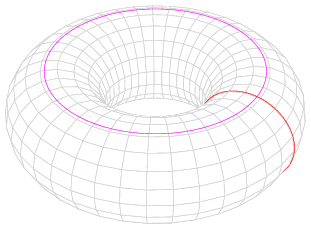While "homology" counts topologically inequivalent noncontractible submanifolds of a sort (for example, the torus has 2 independent loops that may be wound around, namely the two red circles from the picture above whose Cartesian product the torus is, and therefore $$b_1=2$$), there is an equivalent method to obtain the Betti numbers $$b_i$$, namely as the dimensions of the cohomology. Cohomology's elements are not submanifolds. Instead, the elements are differential $$k$$-forms, i.e. completely antisymmetric tensors with $$k$$ indices.

Because $$k$$-forms are "linear forms" acting on $$k$$-cycles ($$k$$-dimensional submanifolds) where the action of the linear form is given by a simple $$k$$-dimensional integral with the natural measure, cohomology and homology are "dual" to each other in the sense of linear algebra (vectors and linear forms on vectors). That's why their dimensions ultimately coincide.

Also, one may Hodge-dualize the differential forms (and choose the by-Laplacian-annihilated representatives of the cohomologies, and this condition is unaffected by the Hodge dualization) which is why for well-behaved manifolds, we also have$b_k = b_{n-k}.$ The list of Betti numbers is left-right-symmetric! For example, the K3 manifold has $(b_0,b_1,b_2,b_3,b_4) = (1,0,22,0,1).$ Because it's even-dimensional, the alternating sum is nonzero, namely $$\chi=24$$ in the case of the K3 surface. But if you had an odd-dimensional manifold, the Betti numbers would look like $$(3,17,17,3)$$ and $$\chi$$ would cancel because each positive term would have a compensating negative term. That's why the Euler characteristic of any odd-dimensional closed manifold is actually zero. This fact also explains why we were only able to write down densities for even dimensions: in odd dimensions, the densities make no sense because the required integral should ultimately vanish, anyway. So you may just define the odd-dimensional Euler densities to vanish by themselves.

Examples of higher-dimensional manifolds and their $$\chi$$

A point, the only connected zero-dimensional manifold, has $$\chi=1$$ as I implicitly said when I described $$\chi$$ as the "regulated number of points". We discussed the Euler characteristic of two-dimensional manifolds in detail (holes, boundaries, crosscaps, and all this stuff). Odd-dimensional closed manifolds have $$\chi=0$$, we just said. So the next interesting case are the 4-real-dimensional manifolds. I have already mentioned that the K3 surface has $$\chi=24$$. The four-torus, much like all tori, has $$\chi=0$$ because it admits a flat metric.

Complex manifolds allow us to split the $$k$$-forms discussed above to $$(p,q)$$-forms that distinguish $$p$$ holomorphic indices and $$q$$ complex conjugate, antiholomorphic indices. We have $$k=p+q$$. Consequently, the cohomologies may be divided to several pieces much like their dimensions, the Betti numbers, which may be written as alternating sums of Hodge numbers:$b_k = \sum_{p,q}^{p+q=k} h_{p,q}.$ For the most interesting six-real-dimensional manifolds, the Calabi-Yau three-folds, the formula for the Euler characteristic reduces to$\chi=\sum_{p,q}(-1)^{p+q} h_{p,q} = 2(h_{1,1}-h_{1,2}).$ All other Hodge numbers except for $$h_{1,1}$$ and $$h_{1,2}$$ are universal, either one or zero, for any Calabi-Yau three-fold. Incidentally, the K3 surface discussed above is also a "complex manifold" and the only decomposition of a Betti number to Hodge numbers that needs an explanation is$22 = b_2 = h_{0,2}+h_{1,1}+h_{2,0} = 1+20+1.$A recent article discussed the Hodge charts, i.e. the Hodge numbers $$h_{1,1}$$ and $$h_{1,2}$$ of (almost?) all the known Calabi-Yau manifolds. The Euler characteristic goes up to $$\pm 960$$ or so. And the mirror symmetry exchanges $$h_{1,1}$$ with $$h_{1,2}$$ (the numbers of two-dimensional and three-dimensional "cycles" get interchanged) which implies that it relates manifolds whose $$\chi$$ are equal to each other up to a sign. In my heuristic terminology above, the mirror symmetry therefore relates members of pairs of manifolds whose number of points are opposite to each other. ;-)

I have already written enough stuff and even the most patient readers have grown bored so let me stop.

That's not the memo, just an expression of a relative exhaustion. :-)snail feedback (17) :

from what i know topology is the hardest area in mathematics.
generally talking if someone had to compare the most difficult and mathematical string theory research with topology research which of the two areas would be considered more difficult?Until about half the article I thought I was reading Gowers' blog.. lol

I was talking with a friend of mine the other day and I came up with an interesting question. Is chirality a property that prevents the universe from having a non orientable topology? I'm not from this field (particles or relativity)

Yes, absolutely, chirality i.e. left-right asymmetry (P symmetry breaking, especially if CP is broken as well) prevents the Universe from having an unorientable topology.

The P-symmetry is what would otherwise guarantee that a left shoe in Boston obeys the same law as a right shoe that made a trip from New York around the world and became another left shoe in Boston. But this symmetry is broken.

So for example, if you send a left-handed neutrino around the world, it becomes a right-handed neutrino, but the latter object doesn't exist (isn't allowed to exist) according to the known laws of physics. Even if you conjectured that it becomes an antiparticle, it won't work because the CP-symmetry is also broken, although by weaker terms than the C-symmetry and P-symmetry themselves.

I don't know how to compare it. Both string theory and topology have lots of layers and subfields - and they also have a significant overlap.

Topology as studied by mathematicians is more rigor-oriented so it's not just about cute schoolkid ways to count topological invariants etc. Its mathematical basis always starts from "topology" in the sense of the rule that says which subsets of a given set (e.g. manifold) are called "open". With this starting point, mathematicians also study lots of pathological, highly discontinuous "topologies" which wouldn't be even considered by physicists because their non-smooth character prevents one from defining a field theory on them, at least so far it looks like that.

At the end, I think that general string theory is more about the creative research while mathematicians' topology is more about a mechanical work, discipline, and avoidance of even the tiniest errors.Do genus and gender have the same root? Just kidding!

I guess this reasoning came from some sin-based intuition that is as dirty so that only Christians may afford it. ;-) But your conclusion is of course 100% right.

http://en.wikipedia.org/wiki/Genus

The term comes from Latin genus "descent, family, type, gender", cognate with Greek: γένος – genos, "race, stock, kin".

http://www.perseus.tufts.edu/hopper/text?doc=Perseus%3Atext%3A1999.04.0057%3Aentry%3D%2321921&redirect=true

Nice didactic post---even easily comprehensible :)

thanksAh, I´ll enjoy this tutorial later at home, where I dont have to fear my nice office mate popping up behind my back and peeking and chuckling at what stuff I`m reading here again ... :-D

What I always like best when reading more mathematical TRF articles is that Lumo alway directly writes in for what cool physics all these things are important and needed :-)tsk tsk . They were not really taught! just not persecuted. Something similar to California mores. The the ionian greek culture which gave us Pythagoras, Thales of Militos , Aristarchos of Samos, and others had an fair respect for women, at least again as in california culture :). See my compilation of women mathematicians in ancient greece http://users.otenet.gr/~avayaki/math.htm .

Dear Anna,

this may be hard for you to convince me otherwise - I learned it at a basic school at my important history teacher. She was also a rather brave anti-communist.

She would repeat that during Olympics, the men would run naked, so that their swinging organs would highlight the beauty of the human body :-), and ... OK, maybe I know this extra information from someone else but he or she had to be comparably trustworthy as my history teacher. :-)

Cheers
LMDid you prepare this post just now all by yourself? Remarkable.

Thanks, Luke, but it's just some rudimentary topology – much of it is the kind of stuff I've been curious about since the (basic) school days. It just seemed appropriate to try to reduce the gap in the understanding of topology after I posted about the Candelas et al. preprint on the Calabi-Yaus - which is obviously above my natural abilities at this point and I would have to work hard to understand everything. So I spent an hour or two last night and decided to postpone the completion to the morning, another hour or two...The last two paragraphs I'll have to reconsider later today(...!). I lost my concentration a bit there and maybe because of that I do not see at present what T means in the definition of the cohomology as a quotient of two boundary operators (?) ...

But dear Lumo, you should NEVER think that there is anything boring about such amazingly powerful and nice articles!
I at least was not bored at all at the end, just a little bit exhausted as you were from writing ;-)

Good night nowHi Lubos,

We also use topology in mine planning. These days, if you want to calculate the optimal size of an open pit, you would use a programme that is based on the Lerch-Grossman algorithm, described in a paper presented in the 1970s by these two IBM mathematicians. The method is referred to as the LG algorithm. It was only the advent of the more powerful - in the sense of doing lots of boring calculations over and over again at a very fast rate - computers that allowed the method to be widely adopted. These days, even humble mining engineers know the basics of topology. It is indeed a very useful branch of mathematics.

On that note, I can remember reading that all the work of Euler is still to be published and that every decade or so a new volume containing new insights from this great man is released. Is this true?

Cool how this fancy science penetrates to useful applications. ;-)

I don't know the extra work waiting to be published. But he was amazing. On the other hand, there are always exaggerated legends in such cases, too. You must ask some genuine Euler-life expert... He lived such a long time ago that it's a bit hard for me to imagine that some of his discoveries still haven't made it to the usual cannon of some math discipline.Hi Lubos,

According to this website, there are four more volumes of Euler's work that are going to be published. He is a truly remarkable man!
http://en.wikipedia.org/wiki/Euler_Commission

I know that Euler used Venn diagrams before Venn, who popularized the use of these diagrams in a singular publication.

By the way, pit optimization is based on Graph Theory, one of the departure points of Topology.

Even a guy like Richard Dawkins refers to topology in that lovely readable book, The Ancestor's Tale to explain evolution. We are all doughnuts as our stomachs are not part of our bodies!